## Estimating the Sum of a Series

Suppose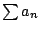is a convergent sequence of positive integers. Let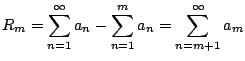which is the error if you approximateusing the first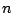terms. From Theorem 6.3.2 we get the following.

Proposition 6.3.8 (Remainder Bound)   Suppose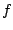is a continuous, positive, decreasing function on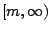andis convergent. Then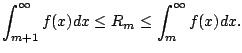Proof. In Theorem 6.3.2 set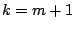. That gives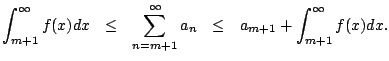But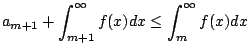sinceis decreasing and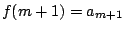.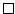Example 6.3.9   Estimate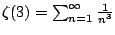using the firstterms of the series. We have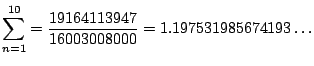The proposition above with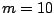tells us that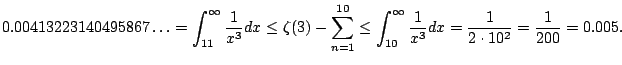In fact,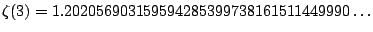and we hvae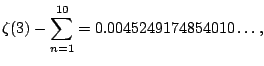so the integral error bound was really good in this case.

Example 6.3.10   Determine if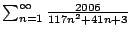convergers or diverges. Answer: It converges, since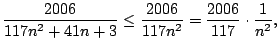and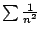converges.

William Stein 2006-03-15